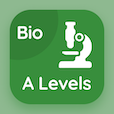Cambridge Online Courses (GCSE)

O Level Physics Quizzes

O Level Physics Quiz PDF - Complete

# Introduction to Waves Quiz MCQ Online p. 180

Practice Introduction to Waves quiz questions and answers PDF, introduction to waves trivia questions 180 to learn online O Level Physics course for online classes. Waves Physics MCQ questions, introduction to waves Multiple Choice Questions (MCQ) for online college degrees. "Introduction to Waves Quiz" PDF eBook: introduction to waves test prep for online college courses.

"A wave is made up of" MCQ PDF: vibrations, air molecules, periodic motions, and oscillations for college entrance exams. Solve waves physics questions and answers to improve problem solving skills for ACT practice test.

## Trivia Quiz on Introduction to Waves MCQs

MCQ: A wave is made up of

air molecules
vibrations
periodic motions
oscillations

### More Quizzes from O Level Physics Course